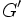# Derived subgroup not is local divisibility-closed in nilpotent group

This article gives the statement, and possibly proof, of the fact that for a group, the subgroup obtained by applying a given subgroup-defining function (i.e., derived subgroup) does not always satisfy a particular subgroup property (i.e., local divisibility-closed subgroup)
View subgroup property satisfactions for subgroup-defining functions$|$ View subgroup property dissatisfactions for subgroup-defining functions

## Statement

It is possible to have a nilpotent group$G$ (in fact, in our example,$G$ is a group of nilpotency class two) such that the [derived subgroup]]$G'$ is not a local divisibility-closed subgroup, i.e., there exists an element of$G'$ that has$n^{th}$ roots in$G$ but no$n^{th}$ roots in$G'$.

## Proof

The simplest examples are:

•$G$ is dihedral group:D8, so$G'$ is the center of dihedral group:D8. The non-identity element of$G'$ has two square roots in$G$ but none in$G'$.
•$G$ is quaternion group, so$G'$ is the center of quaternion group. The non-identity element$-1$ of$G'$ has six square roots in$G$ but none in$G'$.
• For any prime,$G$ is the semidirect product of cyclic group of prime-square order and cyclic group of prime order.$G'$ is a group of prime order, and it lives inside a cyclic subgroup of order$p^2$, hence its non-identity elements have$p^{th}$ roots, none of which are in$G$.